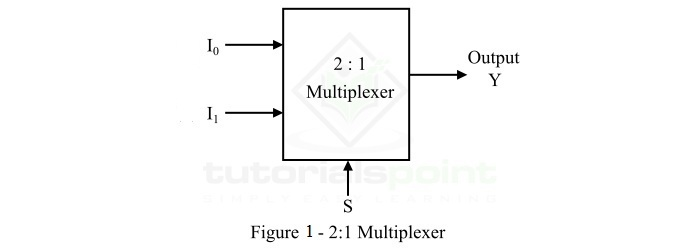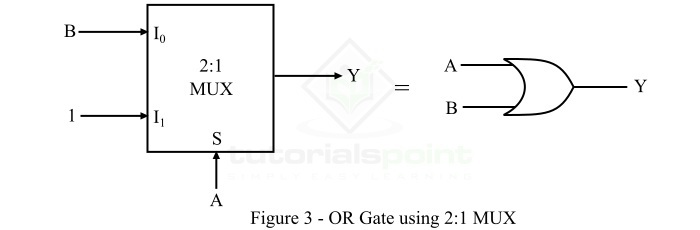# Realizing an OR Gate Using a 2:1 MUX

A multiplexer or MUX is a combinational circuit that accepts several data inputs and allows only one of them to flow through the output line. Multiplexer (MUX) is also known as data selector because it selects one from many.

A MUX consists of 2n data input lines, n select lines, and 1 output line. Since, it converts 2n input lines into 1 output line. Therefore, it is also called many-to-one device.

Depending upon the number of input lines, there are several types of multiplexer present such as 2:1 MUX, 4:1 MUX, 8:1 MUX, etc.

## What is a 2:1 Multiplexer?

The functional block diagram of a 2:1 multiplexer is shown in Figure-1.A 2:1 MUX consists of 2 (21) data input lines designated by I0 and I1, 1 select line designated by S and 1 output line Y. The logic level either 0 or 1 applied to the select line S determines which input data will pass through the output line of the multiplexer.

The operation of the 2:1 MUX can be analyzed with the help of its function table shown below.

Select Line (S) Output (Y)
0 I0
1 I1

From this truth table, we can point out the following,

• If select line S is connected to logic level 0, the data input connected to I0 will pass through the output line Y.

• If select line S is connected to logic level 1, the data input connected to I1 will pass through the output line Y.

Now, let us discuss the basics of OR gate.

## What is an OR Gate?

OR Gate is a basic logic gate that may accept two or more inputs, but gives only one output. The OR gate gives a HIGH or logic 1 state as output if any one of its inputs is in the HIGH or logic 1 state, otherwise, it gives a LOW or logic 0 state as output. Hence, the output of the OR gate is LOW or logic 0 state, only if all its inputs are LOW or logic 0 state. The logic symbol of a two input OR gate is shown in Figure-2.The logic expression of the output of a two input OR gate is given by,

$$Y=A+B$$

Where, the ‘+’ symbol represents the OR operation. It is read as Y is equal to A OR B.

The operation of the OR gate can be analyzed with the help of its function table given below.

Inputs Output
ABY = A + B
000
011
101
111

After getting insights about the basics of 2:1 MUX and OR gate. Let us now discuss the realization of OR gate by using 2:1 MUX.

## Realizing an OR Gate Using a 2:1 Multiplexer

The functional block diagram of a 2:1 multiplexer operating as a two input OR gate is shown in Figure-3.Here, the input B is applied to the input line I0 of the MUX and input A is applied to the select line S. The input line I1 of the MUX is set to logic 1 state.

The operation of the 2:1 MUX as the OR gate can be described as follows −

• When A = 0, the output of the MUX as OR gate is equal to B.

• When A = 1, the output of the MUX as OR gate is equal to logic 1.

The function table of the 2:1 MUX working as an OR gate is given as follows,

A (S) B Y Description
0 0 0

Y = B

When A = 0

0 1 1
1 0 1

Y = 1

When A = 1

1 1 1

Hence, we can analyze it as follows,

$$Y=\bar{A}\cdot \bar{B}\cdot 0+ \bar{A}\cdot B \cdot 0+A\cdot \bar{B} \cdot 0+ A \cdot B \cdot 1=\left ( A+B \right )$$

This is how we can realize an OR gate by using a 2:1 multiplexer.

Updated on: 19-Apr-2023

2K+ Views